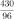# Qn) i) Write the decimal form ofii) Write 0.375 as afraction.iii) A two-digit number divided by another two-digit number gives 5.375. What are the numbers?iv) Write 5.375 as a fraction with numerator a three-digit number and denominator two-digit number. Write more such fractions.

i)=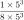= 0.125

ii) 0.375 =x3 =iii) 5.375 = 5 +=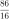iv)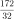;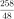;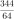;